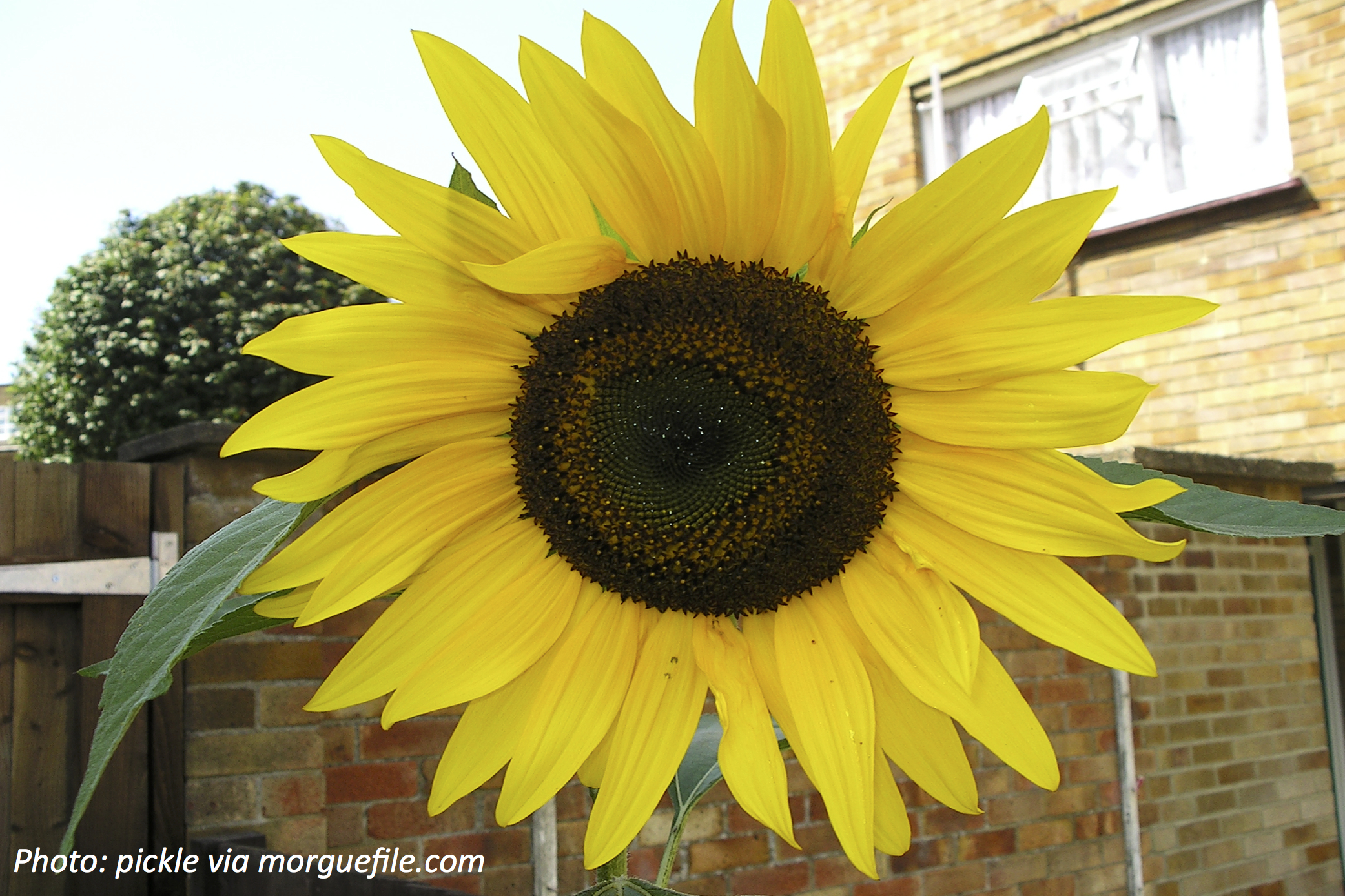# Flowers That CountSpring is here, and that means our friends the flowers are just around the corner. What’s cool is that some flowers do math without even knowing it! Sunflowers always have a certain numbers of petals — only some numbers can work. There’s a special set of numbers where you get each number by adding the 2 numbers before it. You start with 1 and 1, which gives you 2…then 2+1 is 3…then 3 plus that 2 gives you 5…5 plus that 3 gives 8, and so on. They’re called the Fibonacci numbers, and sunflowers always have a Fibonacci number of petals! Let’s see how big that flower can get.

Wee ones: If the first few Fibonacci numbers are 1, 2, 3 and 5, which number did we skip that we would have said if counting?

Little kids: If the last 2 Fibonacci numbers we got were 5 and 8, what’s the next number? (Reminder: You get each number by adding the previous two numbers.) Bonus: If you have a flower with that many petals and another with 5 petals, how many do they have together?

Big kids: Can a sunflower have 25 petals? Bonus: For fun we also have “Tribonacci” numbers, where you add the last 3 numbers to get the next. Since it also starts with 1, 1, and 2, what are the next 3 Tribonacci numbers? See if you can remember all the pieces in your head!

Wee ones: 4.

Little kids: 13. Bonus: 18 petals (13 + 5).

Big kids: No, because after 21 we add 21 + 13, which is 34. Bonus: 4, 7, and 13.

## Pick a Topic

50 States

Animals

Daily Routine

Entertainment

Food

History

Science and Nature

Sports

Vehicles and Transportation